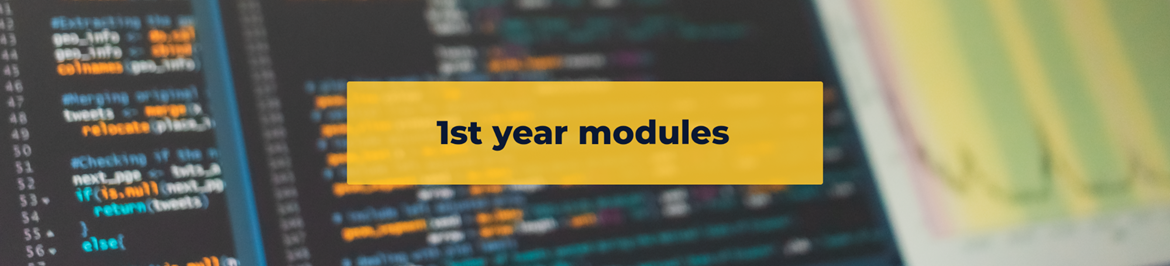# Mathematical Statistics 1

This course consists of the two modules STAS101 and STAS102 which must be passed separately. A student presenting Statistics as a major must do all the respective undergraduate modules. In exceptional cases, the Department may decide to overlook the prerequisite(s) for a module on an individual basis.### STAS101

Probability Theory & Discrete Distribution Theory (15 credits) This module consists of 84 single lectures (6 per week), 28 practicals (2 per week) and 28 tutorials (2 per week) during the first semester.

Pre-requisites

As per faculty requirements.

Content

1. Basic probability concepts: Experiment, sample space and events. Set theory for events.

2. Computing probabilities: Permutations and combinations. Conditional probability. Bayes' Theorem. Functions associated with random variables. Random variables and distributions. Discrete and continuous distributions. Distribution and probability functions. Expected value, variance and moments.

3. Special Distributions: Bernoulli, Binomial, Hypergeometric, Poisson and Negative Binomial.### STAS102

Introduction to Statistical Inference (15 credits) This module consists of 84 single lectures (6 per week), 28 practicals (2 per week) and 28 tutorials (2 per week) during the second semester.

Pre-requisites

At least 40% for STAS101

Content

Maximum likelihood estimation. Least squares estimation. Sufficient statistics. Confidence intervals. Unbiased estimators. Consistent estimators. Efficient estimators. Fisher information. Problems of hypothesis testing. Testing Simple Hypotheses. Statistical tests for means, variances and proportions. Statistical tests for comparing populations.### Statistics 1

Statistics 1 consists of the two modules STAV101 and STAV102 which must be passed separately.

STAV101: Financial Mathematics (12 credits)

This module consists of 56 single lectures (4 per week), 14 practicals (1 per week) and 28 tutorials (2 per week) during the first semester.

Pre-requisites

As per faculty requirements.

Content

Simple and compound interest. Simple and complex annuities. Perpetuities. Applications of annuities and perpetuities. Introduction to linear programming problems. Graphic solution. Computational analysis. Transportation problems. Critical path analysis. Indexes### STAV102: Business Statistics (12 credits)

This module consists of 56 single lectures (4 per week), 14 practicals (1 per week) and 28 tutorials (2 per week) during the second semester.

Pre-requisites

As per faculty requirements.

Content

1. Data: Acquisition, management, patterns and summary measures.

2. Probability: Basic probability concepts, random variables, expected value.

3. Discrete probability distributions: Bernoulli, Binomial and Poisson.

4. Continuous probability distributions: Uniform, Normal and Exponential. Statistical sampling. Point

5. Estimation and the sampling distribution of the sample mean. Interval estimation of population mean. Statistical test for population mean, proportion and variance. Comparisons of two populations. Simple linear regression analysis. Time series analysis.### WSA111: Statistical Methods in Behavioural Sciences (7 credits)

This semester course, WSA111, consists of two 40 minute lectures per week, 40 minutes of practical work per week and two 40 minute tutorials per week.

Content

Elementary data analysis. Statistical measures: median, mean, range, mean deviation and standard deviation. The normal distribution. Normal probability paper and a graphical method to estimate the mean and standard deviation. An understanding of the implications of the law of large numbers and central limit theorem. Standard error and the errors of proportions and differences. Testing of statistical hypotheses, e.g., Student's t-test and Wilcoxon test for the one and two sample cases. Confidence Intervals. The chi-square test for goodness-of-fit. Basic concepts of correlation and simple regression.